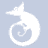quizzes  Forums

# Lesson 2 Quiz

186 played - 11 yrs ago
A review of material covered in Lesson 2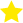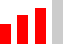30 QUESTIONS
51%1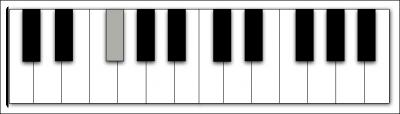What is this note?

3
Which key has one flat, a Bb?

4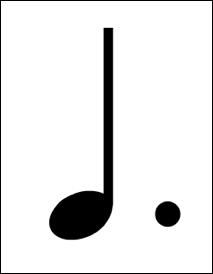In 4/4 time, how many beats would this note get?

5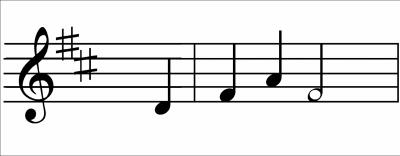What key is this in?

6What are the two sharps built in to this key?

8
What is this symbol?

9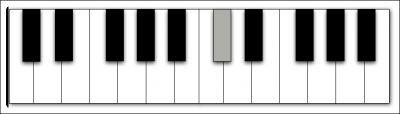What is this note?

10
Which two notes are enharmonic?

11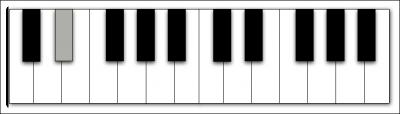What is this note?

12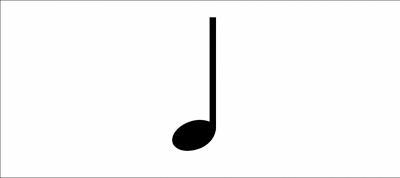What is the name of this note?

13
What is this key signature?

14
What are the three sharps in this key signature?

15
How many eighth notes would fit in one measure of 3/4 time?

17
Which accidental lowers the note a half step?

18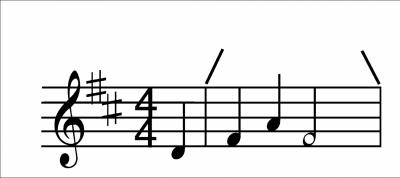What is the distance between two vertical lines, as shown here?

20
Which key is one movement to the right (i. e. one sharp) on the circle of fifths?

21
Which note is enharmonic with F#?

22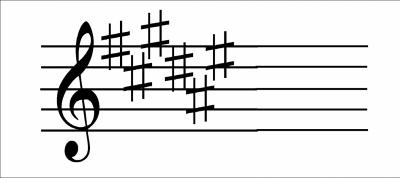What is this key?

23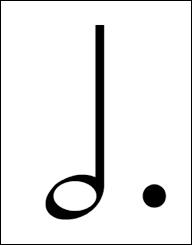In 3/4 time, how many beats would this note get?

25
Which symbol adds the value of two notes?

29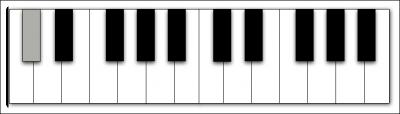What is this note?

30
What is the correct order of the sharps?